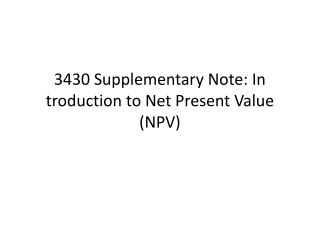DownloadDownload Presentation3430 Supplementary Note: In troduction to Net Present Value (NPV)

3430 Supplementary Note: In troduction to Net Present Value (NPV)

Télécharger la présentation3430 Supplementary Note: In troduction to Net Present Value (NPV)

- - - - - - - - - - - - - - - - - - - - - - - - - - - E N D - - - - - - - - - - - - - - - - - - - - - - - - - - -
Presentation Transcript

1. n CFt ∑ NPV = (1 + R)t t = 0 Net Present Value Sum of the PVs (present value) of all cash flows NOTE: t=0 Initial cost often is CF0 and is an outflow. n CFt ∑ - CF0 NPV = (1 + R)t t = 1

2. NPV – Decision Rule • If NPV is positive, accept the project • NPV > 0 means: • Project is expected to add value to the firm • Will increase the wealth of the owners • NPV is a direct measure of how well this project will meet the goal of increasing shareholder wealth.

3. Sample Project Data • You are looking at a new project and have estimated the following cash flows, net income and book value data: • Year 0: CF = -165,000 • Year 1: CF = 63,120 NI = 13,620 • Year 2: CF = 70,800 NI = 3,300 • Year 3: CF = 91,080 NI = 29,100 • Average book value = \$72,000 • Your required return for assets of this risk is 12%. • This project will be the example for all problem exhibits in this chapter.

4. Computing NPV for the Project • Using the formula: NPV = -165,000/(1.12)0 + 63,120/(1.12)1 + 70,800/(1.12)2 + 91,080/(1.12)3 = 12,627.41

5. Net Present Value Sum of the PVs of all cash flows. << CALCULATOR << EXCEL

6. Rationale for the NPV Method • NPV = PV inflows – Cost NPV=0 → Project’s inflows are “exactly sufficient to repay the invested capital and provide the required rate of return” • NPV = net gain in shareholder wealth • Rule: Accept project if NPV > 0